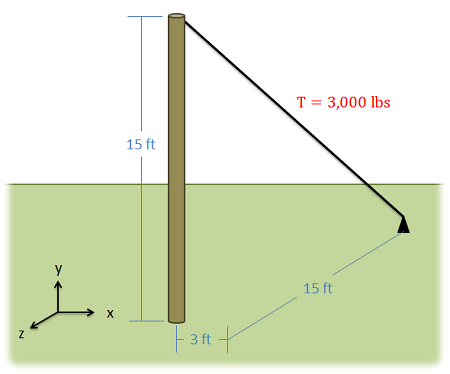﻿ Mechanics Map - Moment About a Point

# Moment About a Point (Vector Calculations)

Given any point on an extended body, if there is a force acting on that body that does not travel through that point, then that force will cause a moment about that point. As discussed on the moments page, a moment is a force's tendency to cause rotation.

## The Vector Method in 2 and 3 Dimensions

An alternative to calculating the moment via scalar quantities is to use the vector method or cross product method. For simple two dimensional problems, using scalar quantities is usually easier, but for more complex problems, using the cross product method is usually easier. The cross product method for calculating moments says that the moment vector of a force about a point will be equal to the cross product of a vector r from the point to anywhere on the line of action of the force and the force vector itself.

 $\vec{M}=\vec{r}\times \vec{F}$

A big advantage of this method is that r does not have to be the shortest distance between the point and the line of action, it goes from the point to any part of the line of action. For any problem, there are many possible r vectors, though because of the way the cross product works, they should all result in the same moment vector in the end.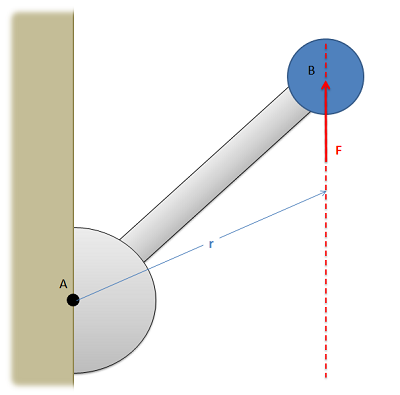The moment vector of the force F about point A will be equal to the cross products of the r vector and the force vector. The r vector is a vector from point A to any point along the line of action of the force.

It is important to note here that all quantities (r, F and M) are vectors. Before you can solve for the cross product, you will need to write out r and F in vector component form. Also, even for two dimensional problems, you will need to write out all three components of the r and F vectors. For two dimensional problems the z components of the r and F vectors will simply be zero, but those values are necessary for the calculations.

The moment vector you get will line up with the axis of rotation for the moment, where you can use the right hand rule to determine if the moment is going clockwise or counterclockwise about that axis.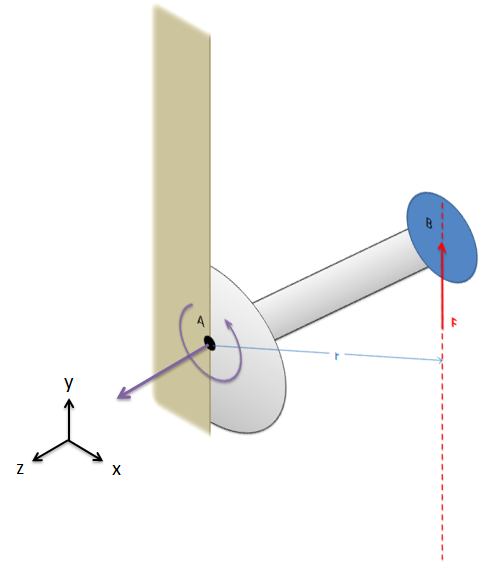The result of r cross F will give us the moment vector. For this two dimensional problem, the moment vector is pointing in the positive z direction. We can use the right hand rule to determine the direction of rotation from the moment (line our right thumb up with the moment vector and our curled fingers will point in the direction of rotation from the moment).

Finally, it is also important to note that cross product, unlike multiplication, is not communicative. This means that the order of the vectors matters, and r cross F will not be the same as F cross r. It is important to always use r cross F when calculating moments.

## Worked Problems:

### Question 1:

What is the magnitude of the moment that the force shown below force exerts about point O?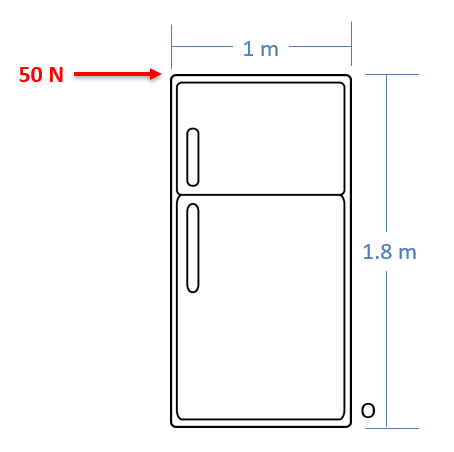### Question 2:

What is the magnitude of the moment that this force exerts…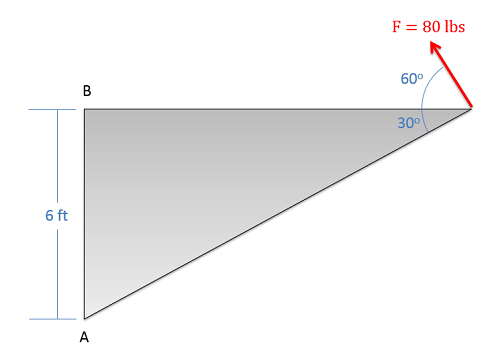### Question 3:

What is the moment that the force shown below exerts about the base of the pole at O? Leave your answer as a moment vector.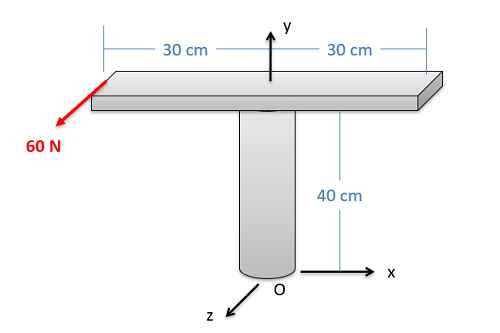### Question 4:

A cable attached to the top of a pole as shown below carries a 3000 lb tension force.

• What is the moment vector this causes about the base of the pole?
• What is the overall magnitude of the moment about the base of the pole?